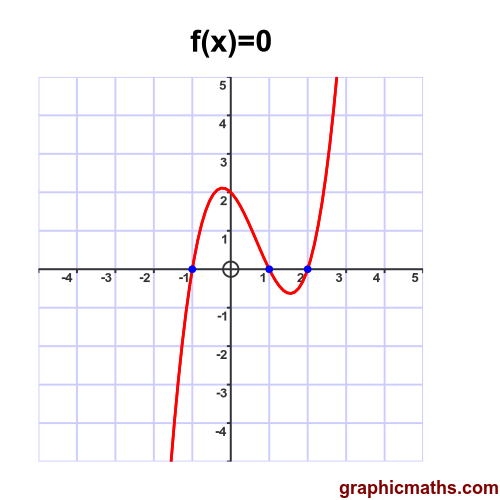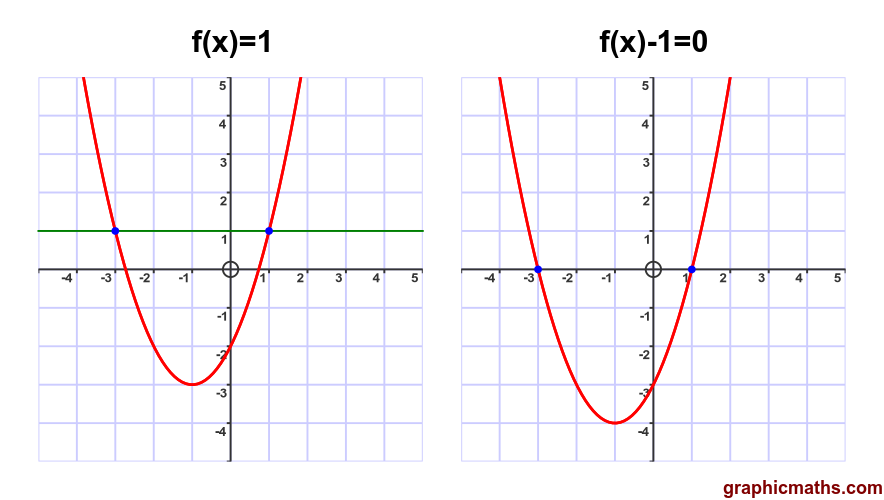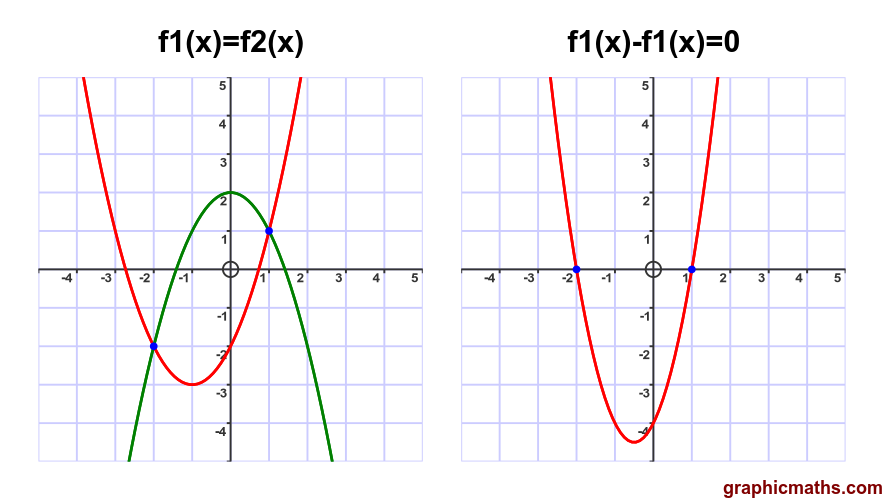# Solving equations numerically

Martin McBride, 2021-03-31
Tags solving equations
Categories numerical methods pure mathematics

In this section we will look at exactly what we mean by solving an equation, why we might need to use numerical methods to solve an equation.

We will only consider functions of one variable, denoted by $f(x)$.

## Solving an equation f(x) = 0

When we solve an equation, we are finding the values of $x$ for which $f(x)$ meets a particular condition. A common type of equation is:

$$f(x)=0$$

In this case we want to find the values of $x$ for which $f(x)$ is zero. For example:

$$x^3 - 2x^2 -x + 2 = 0$$

Here is a graph of this function:In this case the polynomial can be factorised quite easily as:

$$(x + 1)(x - 1)(x - 2) = 0$$

Solutions to this equation are -1, 1, and 2.

## Solving an equation f(x) = c

A second type of equation has the form:

$$f(x)=c$$

Where $c$ is some constant value. This can be rewritten in the form:

$$g(x)=0$$

Where:

$$g(x) = f(x) - c$$

For example the equation:

$$x^2 + 2x - 2 = 1$$

Can be rewritten as

$$x^2 + 2x - 3 = 0$$

As shown in this graph:The left had graph shows the solutions to $f(x)=c$, the right hand graph shows the solutions to $g(x)=0$. In both cases the solutions are -3 and 1.

## Solving an equation f1(x) = f2(x)

A third type of equation has the form:

$$f1(x)=f2(x)$$

In this case we need to find the values of $x$ for which the two formulas have equal values. This can be rewritten in the form:

$$h(x)=0$$

Where:

$$h(x) = f1(x) - f2(x)$$

For example the equation:

$$x^2 + 2x - 2 = 2 - x^2$$

Can be rewritten as

$$2 x^2 + 2x - 4 = 0$$

As shown in this graph:The left had graph shows the solutions to $f1(x)=f2(x)$, the right hand graph shows the solutions to $h(x)=0$. In both cases the solutions are -2 and 1.

## Solving an equation algebraically

In some cases it is possible to find an exact solution for an equation algebraically, for example by rearranging the formula to solve for x:

\begin{align} x^2 = 2\newline x = \sqrt 2 \end{align}

Or factorising:

\begin{align} x^2 + 2x - 3 = 0\newline (x + 3)(x - 1) = 0\newline x = -3, 1 \end{align}

Or using inverse functions

\begin{align} e^x = 3\newline x = \ln 3 \end{align}

Not all equations can be solved in this way. For example, polynomials of degree 5 or higher cannot generally be solved exactly using algebra. In those cases we can use numerical methods.

## Numerical methods

A numerical method is an algorithm that can be used to find an approximate solution to an equation. Most methods use a form of trial and error:

1. Starting with an initial trial value for the solution.
2. Calculate how inaccurate the trial value is (the error).
3. Use a defined method to finding a new trial value that is better than the previous value.
4. Repeat from step 2.

Since this method gives a numerical answer, it is often only an approximation. However, in most cases it is possible to achieve any required degree of precision by repeating the procedure a sufficiently large number of times. This will normally be done by computer, of course, and for many problems an ordinary PC will be able to calculate an answer to 16 significant figures in a fraction of a second.

For simplicity, most numerical methods work with equations of the form $f(x)=0$. As we have seen, it is easy to convert other types of formula to this form.

It is always useful to have a reasonable idea of how many solutions the equation has, and roughly where the solutions are. If possible it is best to sketch the graph before you start.